Latest Banking jobs   »

# Quantitative Aptitude Quiz For SBI PO/Clerk Mains 2023- 10th January

Directions (1-5): In the following questions two quantities are given for each question. Compare the numeric value of both the quantities and answers accordingly.

Q1. Veer invests a certain sum of money at rate of 10% p.a. for 2 years on C.I while Aayush invests Rs.1500 more than that of Veer at the rate of 5% p.a. for 3 years on S.I.
Quantity I: If interest gained by Veer and Aayush are equal, then find Principal invested by Veer.
Quantity II: Rs. 3800
(a) Quantity I > Quantity II
(b) Quantity II > Quantity I
(c) Quantity I ≥ Quantity II
(d) Quantity II ≥ Quantity I
(e) Quantity I = Quantity II or relation can’t be established

Q2. ‘x’ men can do a piece of work in 8 days while ‘y’ women can do the same work in 12 days
Quantity I: Find the time taken by 10 men to complete whole work
Quantity II: Find the time taken by 8 women to complete whole work.
(a) Quantity I > Quantity II
(b) Quantity II > Quantity I
(c) Quantity I ≥ Quantity II
(d) Quantity II ≥ Quantity I
(e) Quantity I = Quantity II or relation can’t be established

Q3. The side of an equilateral triangle is 20 cm.
Quantity I: Find out the radius of circle which is inscribed in the Triangle.
Quantity II: Find out the value of ⅓rd part of its altitude.
(a) Quantity I > Quantity II
(b) Quantity II > Quantity I
(c) Quantity I ≥ Quantity II
(d) Quantity II ≥ Quantity I
(e) Quantity I = Quantity II or relation can’t be established

Q4. Quantity I: What is the speed of second train in m/s, if first train overtakes second train (standing train) at a speed of 35 m/s in 20 sec, while the second train can cross a platform which length is two times more than itself in 20 sec. The length of first train is 250% of length of second train.
Quantity II: What is Speed of train(m/s). The train can cross a 450 m long platform in 25 seconds and crosses a pole in 10 seconds.
(a) Quantity I > Quantity II
(b) Quantity II > Quantity I
(c) Quantity I ≥ Quantity II
(d) Quantity II ≥ Quantity I
(e) Quantity I = Quantity II or relation can’t be established

Q5. Total Surface area of a Cylinder is 968 sq.m. and its height and diameter are in the ratio of 15:14.
Quantity I: Find the difference between total surface area and curved surface area of a Cone, if Cylinder is melted to form a Cone of same height.
Quantity II: Find the surface area of Cylinder if height and radius of the initial Cylinder is interchanged.
(a) Quantity I > Quantity II
(b) Quantity II > Quantity I
(c) Quantity I ≥ Quantity II
(d) Quantity II ≥ Quantity I
(e) Quantity I = Quantity II or relation can’t be established

Directions (6–8): In the given questions, two quantities are given one as ‘Quantity I’ and another as ‘Quantity II’. You have to determine relationship between two quantities and choose the appropriate option.
(a) Quantity I > Quantity II
(b) Quantity I < Quantity II
(c) Quantity I ≥ Quantity II
(d) Quantity I = Quantity II or no relation
(e) Quantity I≤ Quantity II

Q6. Quantity I: Value of T, A, B and C can complete a piece of work in 24, 36 and T days. All three started the work, after 4 days, C left the work and after next 5 days, B also left. Remaining work completed by A in 5.8 days.
Quantity II: Time taken by Q to complete the work alone (in days). P is 20% more efficient than Q who is 25% more efficient than R. If R takes 30 days to complete a piece of work.

Q7. Quantity I: selling of article (in Rs.), Harish bought an article and marked up the price by 60% above its cost price. He gave a discount of Rs.120 such that profit and discount is same.
Quantity II: cost price of article (in Rs.), Anand marked up the price of the article 80% above the cost price and offers a two successive discount of 20% and 10%. He earned a profit of Rs.150.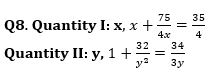Directions (9-11): The following questions are accompanied by two statements, I and II. You have to determine which statements(s) is/are sufficient/necessary to answer the questions.
Q9. Find (P+5R)/(P-R).
I. A number P is first increased by 20% and then decreased by 66⅔% to obtain a number R .
II. Sum of P and R is 1400 and P is 400% more than (R-200).
(a) either I alone or II alone
(b) Only I
(c) only II
(d) neither I nor II
(e) both I and II together

Q10. Find the age of Sohan?
I. Average age of Rohan, Mohan and Sohan is 33 years. Age of Rohan is 20% more than the sum of ages of Mohan and Sohan.
II. Age of Sohan is 62.5% of the difference between age of Rohan and age of Mohan. (Age of Rohan > Age of Mohan > Age of Sohan).
(a) either I alone or II alone
(b) Only I
(c) only II
(d) neither I nor II
(e) both I and II together

Q11. Find the time taken by B alone to finish the work?
I. Efficiency of A is 40% of the efficiency of C and efficiency of B is 33⅓% of efficiency of A, B and C together. Time taken by A and C together to do the work is 6 hours.
II. Difference between the time taken by A alone and C alone to finish the work is 12⅗ hours.
(a) either I alone or II alone
(b) Only I
(c) only II
(d) neither I nor II
(e) both I and II together

Directions (12-15):- Each of the questions consists of two statements I and II. You have to decide which statement/statements is/are sufficient to answer the question. Read the data carefully and answer the following questions.
(a) If statement I alone is sufficient to answer the question
(b) If statement II alone is sufficient to answer the question
(c) If either statement I alone or statement II alone is sufficient to answer the question.
(d) If both statement I and statement II together are not sufficient to answer the question.
(e) If both statement I and statement II together are necessary to answer the question

Q12. Find P?
Statement I: The interest on a certain sum of Rs ‘P’ at 5% per annum for 3 years is Rs 480.
Statement II; The compound interest on Rs ‘P’ at 10% per annum (interest being compounded half yearly) for 1 year is Rs.328.

Q13. Find the time taken by pipe C alone to fill the tank?
Statement I: Pipe A & B together can fill the tank in 30 hours.
Statement II: Pipe B and C together can fill the same tank in 54 hours. All 3 pipes together can fill the tank in 270/11 hours.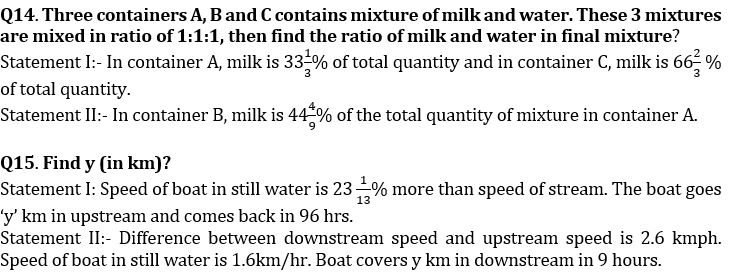Solutions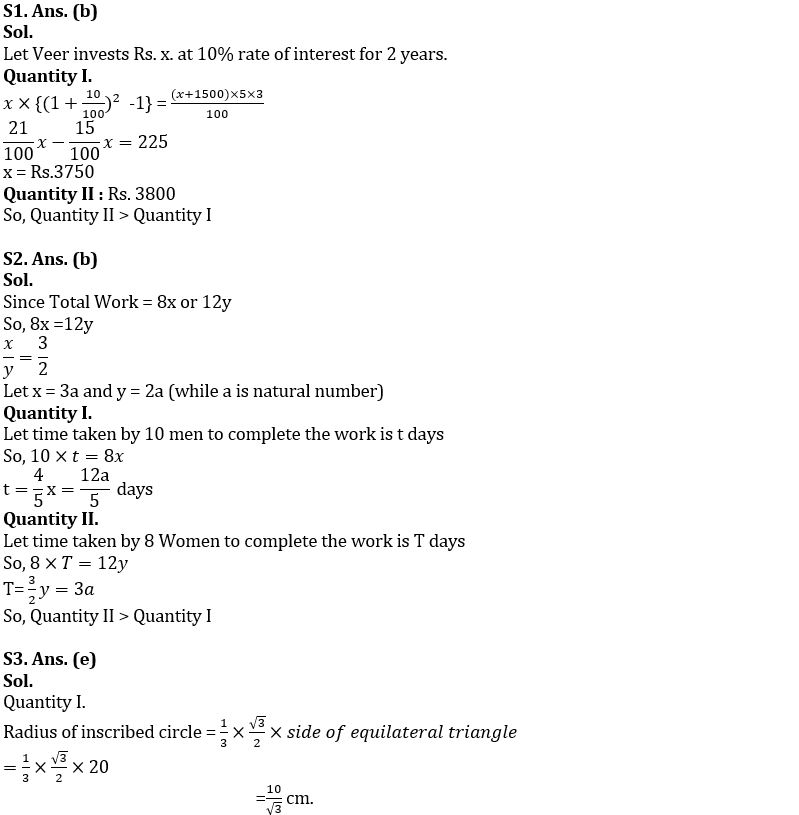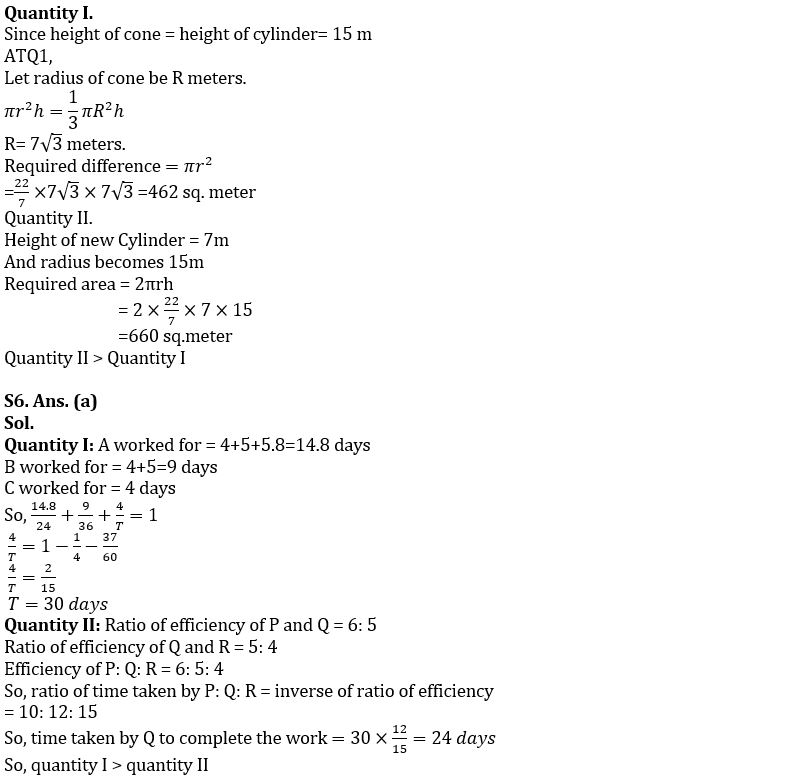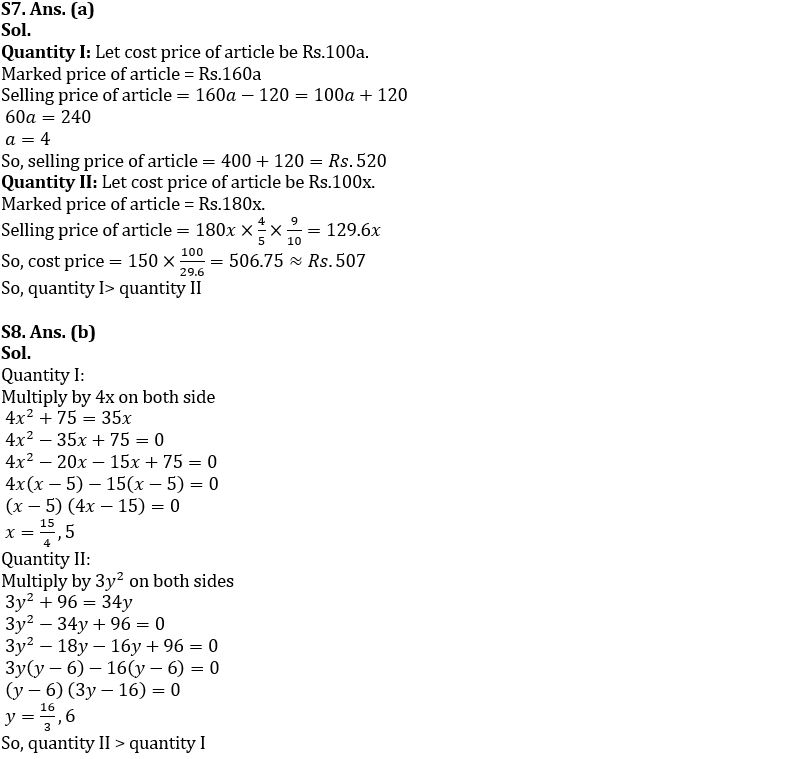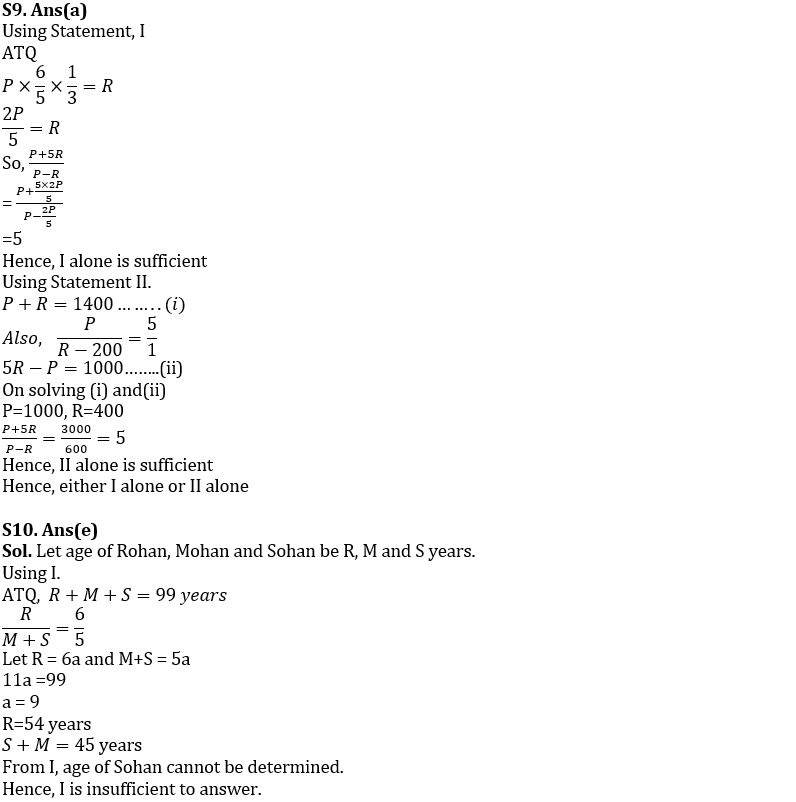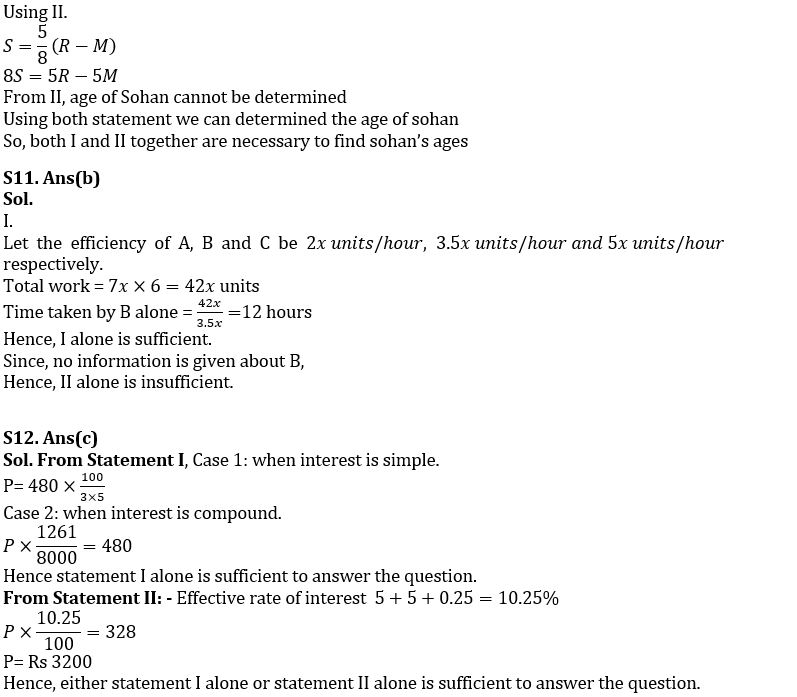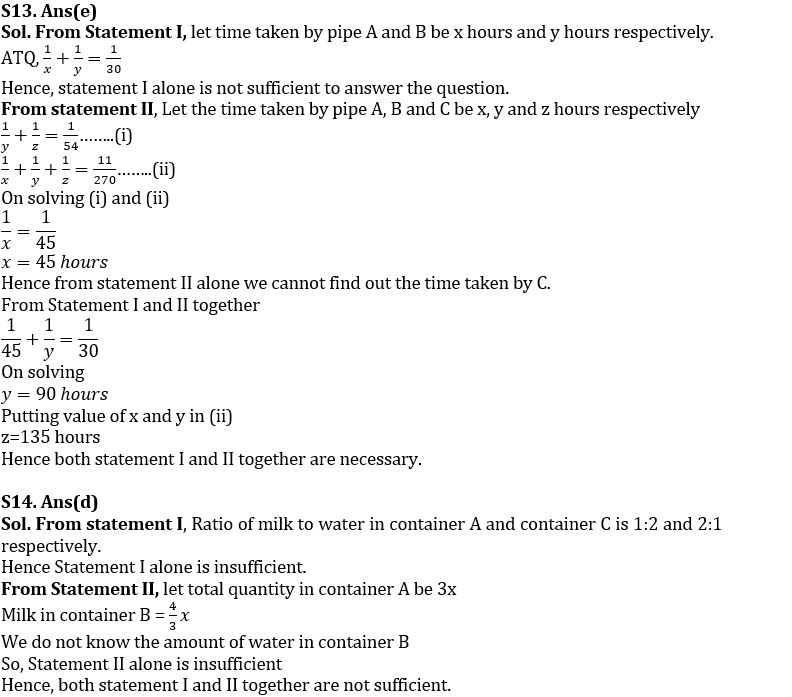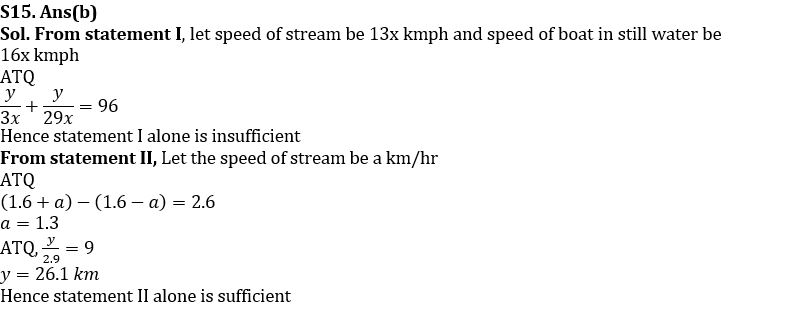## FAQs

### When is the SBI Clerk and PO Mains Exam 2022-23?

SBI Clerk & PO Mains exam are scheduled to be held on 15th & 30th January 2023.

#### Congratulations!Union Budget 2023-24: Free PDF